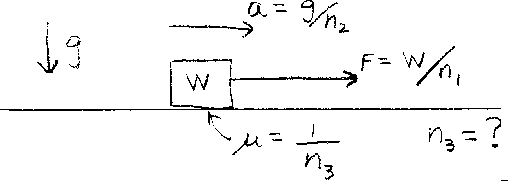Problem B7: A block of weight W can slide on a horizontal surface as shown in the figure above. A force of W/n1 is applied to the right, which causes the block to accelerate with an acceleration equal to g/n2. g is the acceleration due to gravity. If the coefficient of kinetic friction is &mu = 1/n3, then what is n3?n1 = n2 = Input n3:
If you are currently in my class, you can record your grade by entering your name and student ID number (without the leading zeros) below and clicking on "record grade".
 First Name = Last Name = ID = Problem: